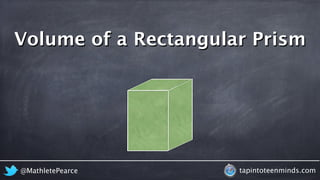Seu SlideShare está sendo baixado. ×

# Deriving the Formula for Volume of a Triangular Prism

Anúncio
Anúncio
Anúncio
Anúncio
Anúncio
Anúncio
Anúncio
Anúncio
Anúncio
Anúncio
AnúncioCarregando em…3
×

1 de 22 Anúncio

# Deriving the Formula for Volume of a Triangular Prism

This video shows the volume of a triangular prism in two ways; the first shows how we can divide a rectangular prism into two (2) pieces by cutting it vertically into two triangular prisms and thus we can do the same to the formula. We also look at the area of the base and how we get the area of a triangle to help guide us to the volume.

This video shows the volume of a triangular prism in two ways; the first shows how we can divide a rectangular prism into two (2) pieces by cutting it vertically into two triangular prisms and thus we can do the same to the formula. We also look at the area of the base and how we get the area of a triangle to help guide us to the volume.

Anúncio
Anúncio

### Deriving the Formula for Volume of a Triangular Prism

1. 1. Where Does The Volume of a Triangular Prism Come From? @MathletePearce tapintoteenminds.com
2. 2. Volume of a Rectangular Prism @MathletePearce tapintoteenminds.com
3. 3. Volume of a Rectangular Prism @MathletePearce tapintoteenminds.com
4. 4. Volume of a Rectangular Prism A = lw @MathletePearce tapintoteenminds.com
5. 5. Volume of a Rectangular Prism A = lw V = lwh h @MathletePearce tapintoteenminds.com
6. 6. Volume of a Triangular Prism V = lwh h A = lw 2 @MathletePearce tapintoteenminds.com
7. 7. 2 Volume of a Triangular Prism V = lwh h A = 2 lw @MathletePearce tapintoteenminds.com
8. 8. Volume of a Triangular Prism V = lwh h 2 A = lw 2 @MathletePearce tapintoteenminds.com
9. 9. Volume of a Triangular Prism H V = H 2 @MathletePearce tapintoteenminds.com lw 2 bh A = bh
10. 10. Volume of a Triangular Prism H V = H 2 @MathletePearce tapintoteenminds.com lw 2 bl A = bl
11. 11. Volume of a Triangular Prism h V = h 2 @MathletePearce tapintoteenminds.com lw 2 bl A = bl
12. 12. Volume of a Triangular Prism h V = h 2 @MathletePearce tapintoteenminds.com lw 2 bl A = bl
13. 13. Volume of a Triangular Prism @MathletePearce tapintoteenminds.com lw
14. 14. Volume of a Rectangular Prism A = lw @MathletePearce tapintoteenminds.com
15. 15. 2 lw Triangular A = Volume of a Prism @MathletePearce tapintoteenminds.com
16. 16. 2 Volume of a Triangular Prism lw A = length width @MathletePearce tapintoteenminds.com
17. 17. Volume of a Triangular Prism lw A = 2 length width @MathletePearce tapintoteenminds.com
18. 18. Volume of a Triangular Prism lw A = height base 2 @MathletePearce tapintoteenminds.com
19. 19. Volume of a Triangular Prism A = bh height base 2 @MathletePearce tapintoteenminds.com
20. 20. blh Volume of a Triangular Prism A = height bh 2 V = bh H 2 @MathletePearce tapintoteenminds.com
21. 21. Volume of a Triangular Prism A = height bl 2 V = bl h 2 @MathletePearce tapintoteenminds.com bhH
22. 22. Volume of a Triangular Prism A = height bl 2 V = bl h 2 @MathletePearce tapintoteenminds.com